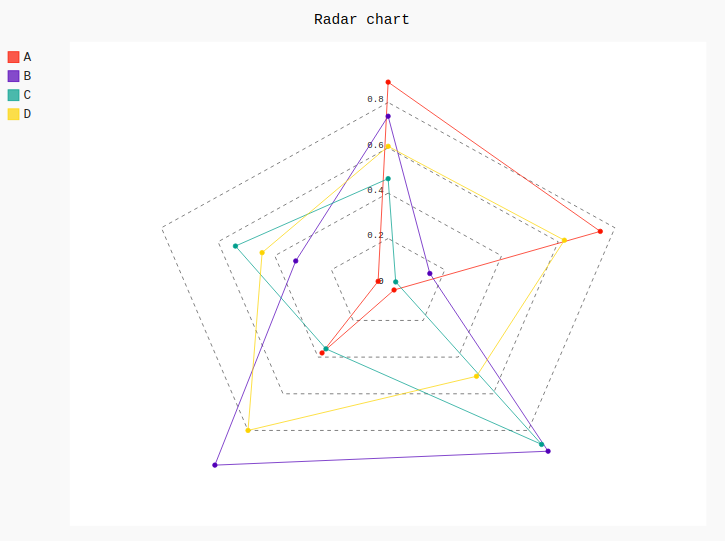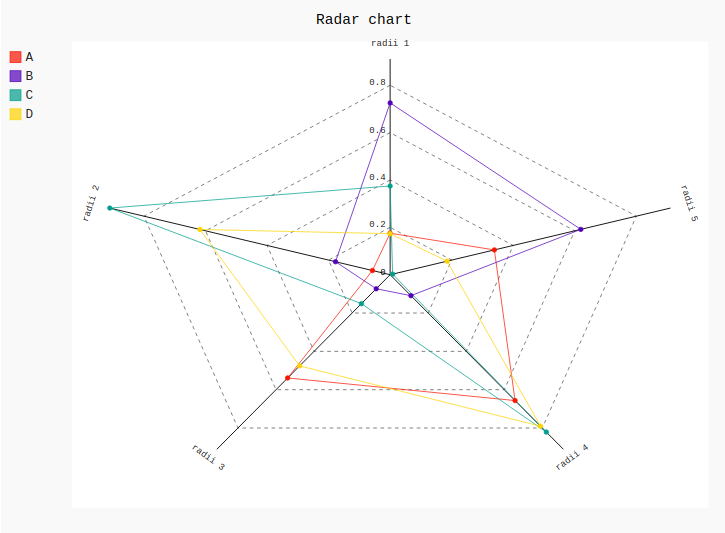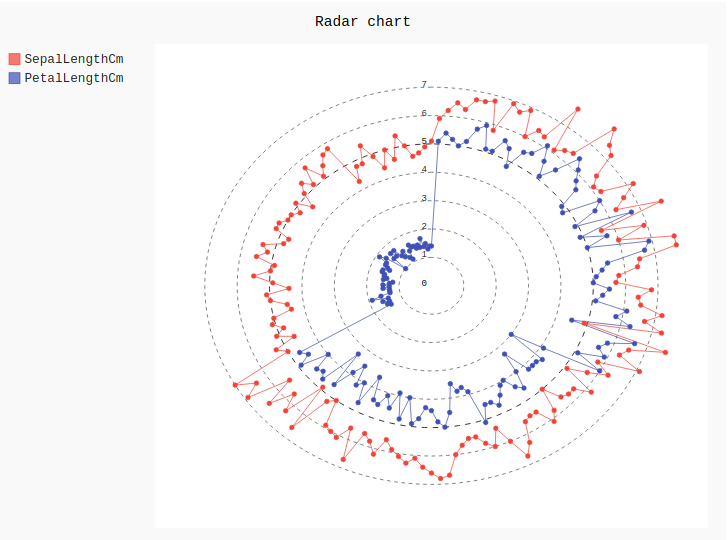Skip to content
Related Articles
Radar chart in pygal
• Last Updated : 10 Jul, 2020

Pygal is a Python module that is mainly used to build SVG (Scalar Vector Graphics) graphs and charts. SVG is a vector-based graphics in the XML format that can be edited in any editor. Pygal can create graphs with minimal lines of code that can be easy to understand.

## Radar Chart

The radar chart is a chart or plot that consists of a sequence of equiangular spokes, called radii, with each spoke representing one of the variables. A radar chart is basically a graphical method of displaying data in the form of a two-dimensional chart of three or more quantitative variables that are represented on the axes starting from the same point. Radar charts are MORE helpful for small-to-moderate-sized multivariate data sets. It can be created using the Radar() method.

Syntax:

```radar_chart = pygal.Radar()
```

Example 1:

## Python3

 `# importing pygal``import` `pygal``import` `numpy`` ` ` ` `# creating the chart object``radar_chart ``=` `pygal.Radar()`` ` `# naming the title``radar_chart.title ``=` `'Radar chart'`` ` `# Random data``radar_chart.add(``'A'``, numpy.random.rand(``5``))``radar_chart.add(``'B'``, numpy.random.rand(``5``))``radar_chart.add(``'C'``, numpy.random.rand(``5``))``radar_chart.add(``'D'``, numpy.random.rand(``5``))`` ` `radar_chart`

Output:Example 2:

## Python3

 `# importing pygal``import` `pygal``import` `numpy`` ` ` ` `# creating the chart object``radar_chart ``=` `pygal.Radar()`` ` `# naming the title``radar_chart.title ``=` `'Radar chart'`` ` `radar_chart.x_labels ``=` `[``'radii 1'``, ``'radii 2'``,``                        ``'radii 3'``, ``'radii 4'``,``                        ``'radii 5'``]`` ` `# Random data``radar_chart.add(``'A'``, numpy.random.rand(``5``))``radar_chart.add(``'B'``, numpy.random.rand(``5``))``radar_chart.add(``'C'``, numpy.random.rand(``5``))``radar_chart.add(``'D'``, numpy.random.rand(``5``))`` ` `radar_chart`

Output:Example 3: Using Iris Dataset

## Python3

 `# importing pygal``import` `pygal``import` `pandas`` ` ` ` `# creating the chart object``radar_chart ``=` `pygal.Radar()`` ` `# naming the title``radar_chart.title ``=` `'Radar chart'`` ` `df ``=` `pandas.read_csv(``'Iris.csv'``)`` ` `radar_chart.add(``"SepalLengthCm"``, df[``'SepalLengthCm'``])``radar_chart.add(``"PetalLengthCm"``, df[``'PetalLengthCm'``])``radar_chart`

Output:Attention geek! Strengthen your foundations with the Python Programming Foundation Course and learn the basics.

To begin with, your interview preparations Enhance your Data Structures concepts with the Python DS Course. And to begin with your Machine Learning Journey, join the Machine Learning – Basic Level Course

My Personal Notes arrow_drop_up